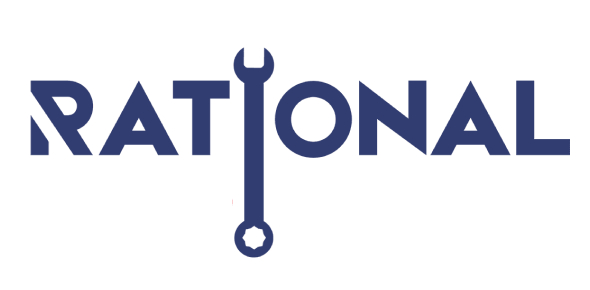# Rational And Irratonal Square Roots

10 Questions | Total Attempts: 186Settings• 1.
The square root of 64 is irrational.
• A.

True

• B.

False

• 2.
The square root of 34.6 is rational.
• A.

True

• B.

False

• 3.
The square root of 36 is rational.
• A.

True

• B.

False

• 4.
The square root of 81 is rational.
• A.

True

• B.

False

• 5.
The square root of 82 is rational.
• A.

True

• B.

False

• 6.
The square root of 72.6 is irrational.
• A.

True

• B.

False

• 7.
The square root of 25 is rational.
• A.

True

• B.

False

• 8.
The square root of 50 is irrational.
• A.

True

• B.

False

• 9.
The square root of 100 is irrational.
• A.

True

• B.

False

• 10.
The square root of 49 is irrational.
• A.

True

• B.

False

Related TopicsBack to top